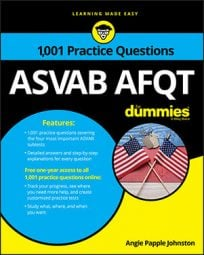##### ASVAB AFQTWhen you run into a problem on the Mathematics Knowledge subtest on the ASVAB that deals with quadratic equations, you can use different ways to solve it: by factoring, completing the square, or using the quadratic formula.

The quadratic formula for equations in the form of ax2 + bx + c = 0 looks like this:

## Practice questions

1. Express as a quadratic equation: 2x2 – 8x – 4 = 3xx2

A. x2 – 11x – 4 = 0 B. 3x2 – 11x – 4 = 0 C. 3x2 – 5x – 4 = 0 D. 3x2 – 11x – 3 = 0

2. Solve for x: x2 – 3x = 0

1. The correct answer is Choice (B).

A quadratic equation has an x2 term. When the equation is in standard form, both sides equal 0. Put all the terms on the same side, set the other side equal to 0, and combine like terms:

2. The correct answer is Choice (A).

A quadratic equation's standard form is ax2 + bx + c = 0. Although this equation shows only two terms, x2 and –3x, it's still quadratic because there's an x2 term (you can assume c = 0).

Solve this quadratic equation by factoring:

Set each factor equal to 0; then solve:

The solutions are x = 0 and x = 3.